# 分数乘法的意义，人教版六年级上册数学（1）分数乘法解析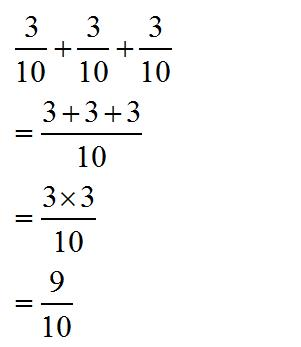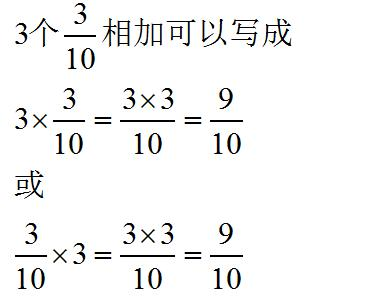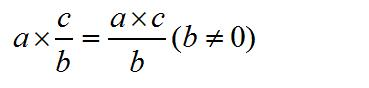12的一半是多少？

12÷2=6

12的一半就是把12平均分成2份，然后占其中的一份，也就是求12的1/2是多少？

12×1/2=（12×1）/2=6

15×1/3=5

1/2的1/6是多少？

1/2就是把单位1平均分成2份取其中的1份，

1/2的1/6就是把1/2平均分成6份，取其中的1份，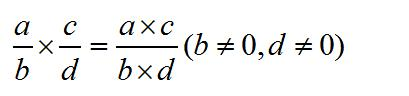2.4m的3/4是多少m？

（1）

2.4×3/4=12/5×3/4=（12×3）/（5×4）=9/5（m）

（2）

2.4×3/4=2.4×0.75=1.8（m）

（3）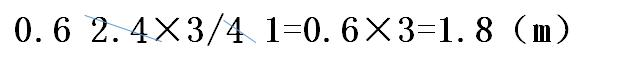（1）可以把小数化成分数，然后按照分数乘分数的方法计算。（要把带分数化成假分数计算，不能用带分数计算，能约分的要约成最简分数。）

（2）可以把分数化成小数，然后按照小数乘小数的方法计算。（分数化为小数位无限小数和循环小数时不能用此方法。）

（3）如果小数和分数的分母是因数和倍数的关系时，可以小数和分数的分母直接约分，然后再计算。

（1）长方形的周长=长×2+宽×2

2/5×2+1/4×2

=4/5+1/2

=8/10+5/10

=13/10（dm）

（2）长方形的周长=（长+宽）×2

（2/5+1/4）×2

=（8/20+5/20）×2

=13/20×2

=13/10（dm）

（2/5+1/4）×2

=2/5×2+1/4×2

=4/5+1/2

=8/10+5/10

=13/10（dm）

（1/2×3/5）×2/5=1/2×（3/5×2/5）

（2/5+1/4）×2=2/5×2+1/4×2

41×2>41

41×1=41

41×0.1<41

a×b（b>1）>a，（a不为0）

a×b（b=1）=a，（a不为0）

a×b（b<1）<a，（a、b不为0）

2/5×2>2/5

2/5×1=2/5

2/5×1/2<2/5

a/b×c（c>1）>a/b，（a、b不为0）

a/b×c（c=1）=a/b，（a、b不为0）

a/b×c（c<1）<a/b，（a、b、c不为0）

（1）

1/2×1/4=1/8

480×1/8=60（m²）

（2）

480×1/2=240（m²）

240×1/4=60（m²）

480×1/2×1/4

=240×1/4

=60（m²）

480×（1/2×1/4）

=480×1/8

=60（m²）

（1）把小明跳的总数看成单位“1”，小亮比小明多跳了1/6

120的7/6：120×7/6=140（下）

120×（1+1/6）

=120×7/6

=140（下）

（2）先算出小亮比小明多跳了多少下

120×1/6=20（下）

120+20=140（下）

120+120×1/6

=120+20

=140（下）

（1）

15×（1-1/3）

=15×2/3

=10（岁）

（2）

15-15×1/3

=15-5

=10（岁）","content_hash":"61986b3f